# Detect orientation of a cube with openCV

by Daniel Pollithy — on  ,  ,  ,Setup: There are 8 cameras positioned around a cube at which they are looking (ref: Initial setup). Every face of the cube is colored differently.

Scope: Spot the location of every camera by analyzing its rgb stream.

Example: Camera No. 1 sees the blue, red and green face and therefore has to be south-bottom.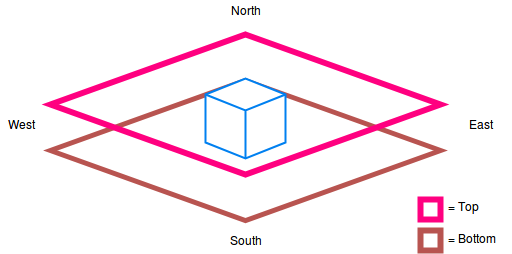The idea: If I could remove everything else except for the cube in the rgb stream, then I could easily match colored faces and from there derive the orientation of the cube.

## First try

I made the asumption that the colors of the cube’s faces are unique in the image. After crafting a 6cm cube from cardboard and painting it (see image cube) I got to work with openCV 2.6.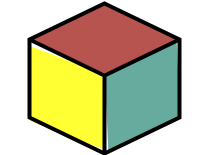``````import cv2
import numpy as np
``````

The background of the image should be noisy because I wanted to build a robust solution. So I found a real life object similar to a cube: a printer.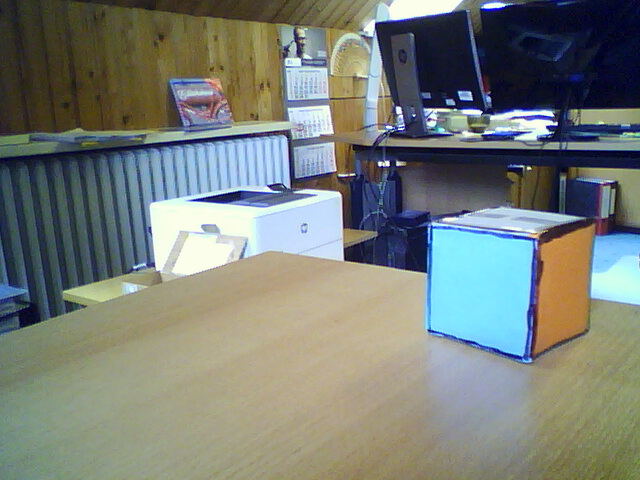### Isolating the cube

1. Create an all black mask
3. Add all areas filled with the colors of the cube to the mask
4. Remove areas of the combined mask which are too small to be a cube (for example a line)

``````# get the dimensions of the input image
width = img.shape[1::-1]
height = img.shape[1::-1]
# create a black image with the same dimensions
# create a white image with the same dimensions
all_white = np.ones((width,height,3), np.uint8)
all_white[:] = (255, 255, 255)
# change the colorspace to rgb
``````

``````# blur the image
img_blurred = cv2.medianBlur(img, 17)
# remove noise with bilateral filter
img_noise_removed = cv2.bilateralFilter(img_blurred, 9,75,75)

# convert to grayscale
img_gray = cv2.cvtColor(noise_removal,cv2.COLOR_RGB2GRAY)
# use adaptive gaussian treshold to convert the image to black and white
cv2.THRESH_BINARY, 11, 2)

# canny edge detection
canny_image = cv2.Canny(thresh_image,250,255)
# dilate to strengthen the edges
kernel = np.ones((9,9), np.uint8)
dilated_image = cv2.dilate(canny_image,kernel,iterations=1)
``````

First define the hsb colors:

``````hsb_colors = {
'blue':   ([ 37, 85, 75], [150, 230, 255]),
'orange': ([  1, 155, 127], [ 38, 255, 255]),
'green': ([ 30,  60, 70], [ 90,  180, 255]),
'red': 	  ([  0, 151, 100], [ 30, 255, 255])
}
``````

Now loop over the colors, find the areas and add them to the mask:

``````for key, (lower, upper) in hsb_colors.items():
# create NumPy arrays from the boundaries
lower = np.array(lower, dtype = "uint8")
upper = np.array(upper, dtype = "uint8")

# find the colors within the specified boundaries and apply the mask
``````

#### Remove small areas

Bring the lines and areas together:

``````combined = cv2.add(dilated_image, blank_mask)
``````

Now dilate and erode to strengthen the cubes mask and remove lines and other noise.

``````# bring the lines and areas closer together (merge them)
kernel = np.ones((11,11), np.uint8)
combined_dilated = cv2.dilate(combined, kernel, iterations=1)

# remove everything smaller than 71x71
kernel = np.ones((71,71), np.uint8)
combined_eroded = cv2.erode(combined_dilated, kernel, iterations=1)

# increase everything in size that is left
kernel = np.ones((91,91), np.uint8)
combined_area = cv2.dilate(combined_eroded, kernel, iterations=1)

final_img = np.zeros((width,height,3), np.uint8)
final_img = cv2.bitwise_and(img, img, mask = combined_area)
``````

Show the result:

``````cv2.namedWindow("final cube image", cv2.WINDOW_NORMAL)
cv2.imshow("final cube image", final_img)
``````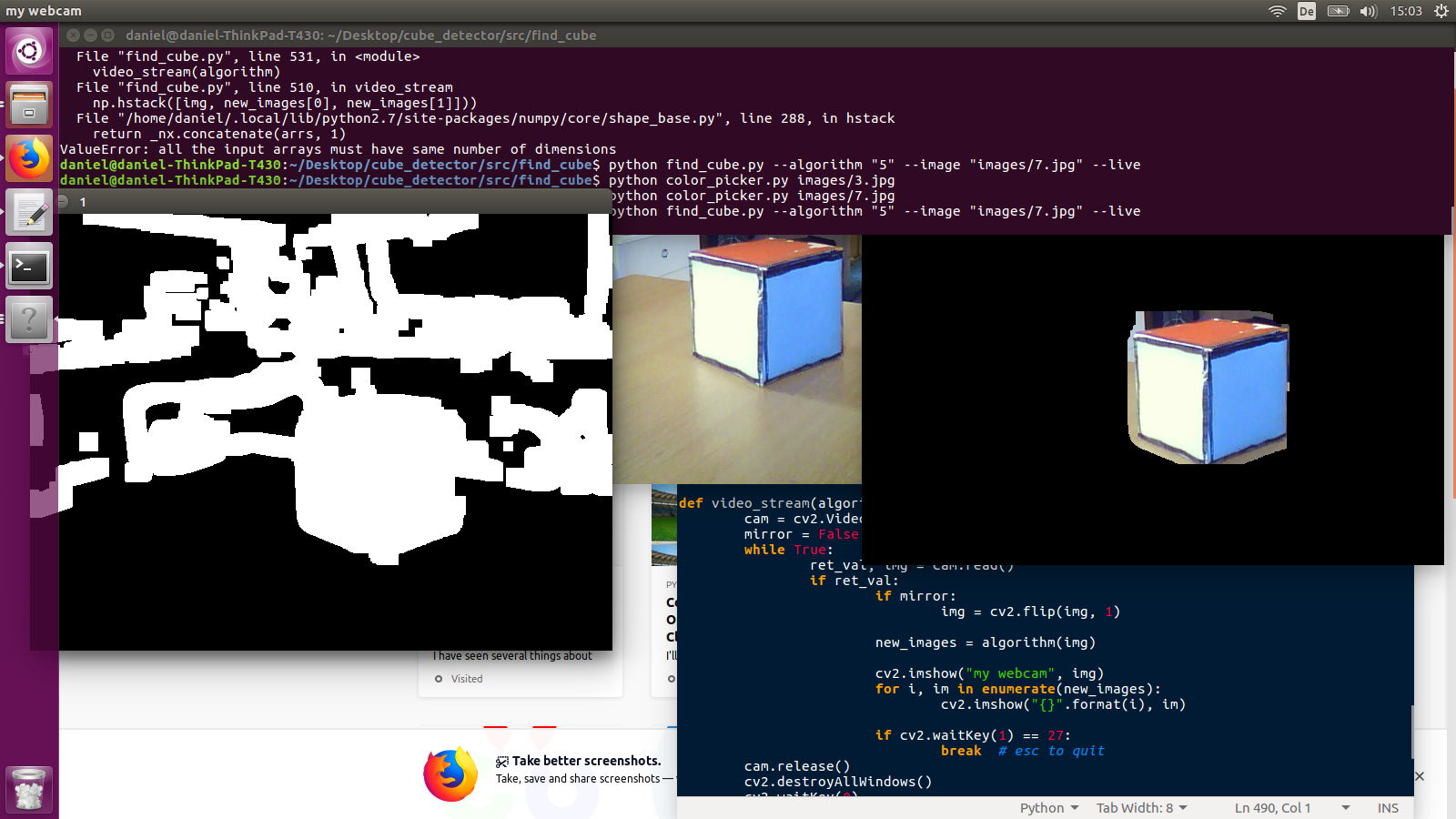### Does the approach work?

Looking at the last screenshot one could assume that this approach works. Well… Looking at the cube from the correct angle with the perfect amount of light. This works. But if the light or the angle changes the color ranges are not large enough. When I tried to adjust them too many colors were in the range and a lot of areas in the image were big enough to overcome the fourth described stage. The color problem is visible in the next image.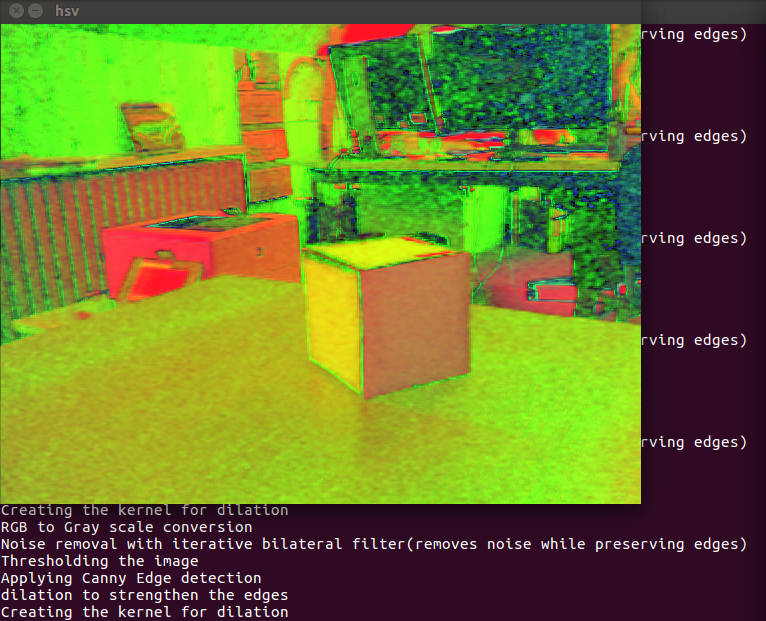So I started all over again with a new idea.

## Second try

My second approach is to use the a priori knowledge about cubes to find them by their edges not the size of masks or the color of faces.

1. Find the most important edges
2. Find all perpendicular lines that are equally long and close to each others
3. Connect the upper ends of lines and lower ends of lines
4. Cluster the ends of lines with DBSCAN to find the edges of the cube

### Find the most important edges

``````# do the same preprocessing as before
img_gray = cv2.cvtColor(img, cv2.COLOR_RGB2GRAY)
noise_removal = cv2.bilateralFilter(img_gray, 9,75,75)
cv2.THRESH_BINARY, 11, 2)

# this dilate and erode section is not optimal and
# the sizes of the kernels is the result multiple attempts
kernel = np.ones((10,1), np.uint8)
dilated_thresh_image = cv2.dilate(thresh_image, kernel, iterations=1)

kernel = np.ones((10,1), np.uint8)
dilated_thresh_image = cv2.erode(dilated_thresh_image, kernel, iterations=1)

kernel = np.ones((5,5), np.uint8)
dilated_thresh_image = cv2.erode(dilated_thresh_image, kernel, iterations=1)

kernel = np.ones((20,1), np.uint8)
dilated_thresh_image = cv2.dilate(thresh_image, kernel, iterations=1)

kernel = np.ones((25,1), np.uint8)
dilated_thresh_image = cv2.erode(dilated_thresh_image, kernel, iterations=1)

kernel = np.ones((5,5), np.uint8)
dilated_thresh_image = cv2.erode(dilated_thresh_image, kernel, iterations=1)

# invert the black and white image for the LineDetection
inverted_dilated_thresh_image = cv2.bitwise_not(dilated_thresh_image)

img2 = img.copy()
``````

### Find perpendicular lines

Find all lines:

``````# Control the lines we want to find (minimum size and minimum distance between two lines)
minLineLength = 100
maxLineGap = 80

# Keep in mind that this is opencv 2.X not version 3 (the results of the api differ)
lines = cv2.HoughLinesP(inverted_dilated_thresh_image,
rho = 1,
theta = 1 * np.pi/180,
lines=np.array([]),
threshold = 100,
minLineLength = minLineLength,
maxLineGap = maxLineGap)
``````

Now select the perpendiucalar lines:

``````# storage for the perpendiucalar lines
correct_lines = np.array([])

if lines is not None and lines.any():
# iterate over every line
for x1,y1,x2,y2 in lines:

# calculate angle in radian (if interesten in this see blog entry about arctan2)
angle = np.arctan2(y1 - y2, x1 - x2)
# convert to degree
degree = abs(angle * (180 / np.pi))

# only use lines with angle between 85 and 95 degrees
if 85 < degree < 95:
# draw the line on img2
cv2.line(img2,(x1,y1),(x2,y2),(0,255,0),2)

# correct upside down lines (switch lower and upper ends)
if y1 < y2:
temp = y2
y2 = y1
y1 = temp
temp = x2
x2 = x1
x1 = temp

# store the line
correct_lines = np.concatenate((correct_lines, np.array([x1,y1,x2,y2], \
dtype = "uint32")))

# draw the upper and lower end on img2
cv2.circle(img2, (x1,y1), 2, (0,0,255), thickness=2, lineType=8, shift=0)
cv2.circle(img2, (x2,y2), 2, (255,0,0), thickness=2, lineType=8, shift=0)
``````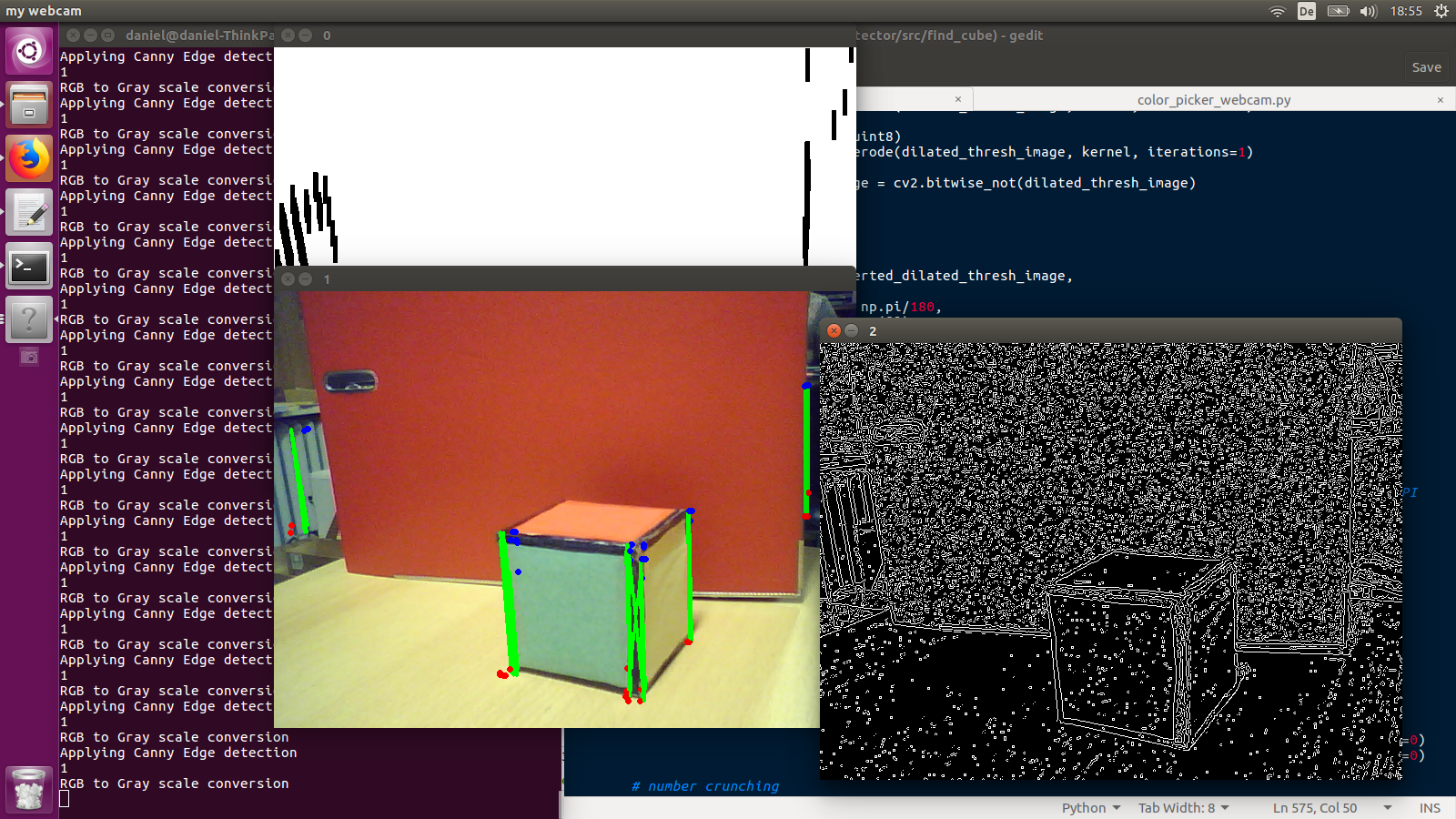### Connect the upper ends of lines and lower ends of lines

Our a priori knowledge about the given cube is that the vertical edges will be perpendicular. But we can’t make such an asumption about the the horizontal edges.

What we can do, is connect the upper ends of line A with other upper ends of lines that are in range of A. In range means for example that the euclidean distance is maximum the length of the line A.

Additionally we only connect ends of lines that have a similar length.

``````# lots of storage for findings
squares = np.array([])
lower_points = np.array([])
upper_points = np.array([])
top_lines = np.array([])
bottom_lines = np.array([])
areas = np.array([])

# reshape the numpy array to a matrix with four columns
correct_lines = correct_lines.reshape(-1, 4)

for a_x1, a_y1, a_x2, a_y2 in correct_lines:
line_length = np.linalg.norm(np.array([a_x1, a_y1])-np.array([a_x2, a_y2]))

for b_x1, b_y1, b_x2, b_y2 in correct_lines:
line_length_b = np.linalg.norm(np.array([b_x1, b_y1])-np.array([ b_x2, b_y2]))

# O(n^2)
# Compare all lines with each others

# only those with similar length
if 0.9 > max(line_length, line_length_b)/min(line_length, line_length_b) > 1.1:
continue

# distance between the top points of the lines
dist = np.linalg.norm(np.array([ a_x1, a_y1 ]) - np.array([b_x1, b_y1]))

# lines that are too close to eachs others (or even the same line) excluded
# also exclude those too distant
if 20 < dist < line_length:

# distance between lower points
dist = np.linalg.norm(np.array([ a_x2, a_y2 ]) - np.array([b_x2, b_y2]))

# if the lower points also match
if 20 < dist < line_length:
# NOW: create the line between the uppder and lower ends
top_lines = np.concatenate((top_lines, np.array([a_x1,a_y1,b_x1,b_y1], \
dtype = "uint32")))
angle_top_line = np.arctan2(int(a_y1) - int(b_y1), int(a_x1) - int(b_x1))
degree_top_line = abs(angle_top_line * (180 / np.pi))

bottom_lines = np.concatenate((bottom_lines, np.array([a_x1,a_y1,b_x1,b_y1], \
dtype = "uint32")))
angle_bottom_line = np.arctan2(int(a_y1) - int(b_y1), int(a_x1) - int(b_x1))
degree_bottom_line = abs(angle_bottom_line * (180 / np.pi))

# hack around 0 degree
if degree_top_line == 0 or degree_bottom_line == 0:
degree_top_line += 1
degree_bottom_line += 1

# if the upper and lower connection have an equal angle
# they are interesting corners for a cube's face
if 0.8 > max(degree_top_line, degree_bottom_line)/min(degree_top_line,
degree_bottom_line) > 1.2:
print("too much difference in line degrees")
continue

# draw the upper line and store its ends
cv2.line(img2, (int(a_x2), int(a_y2)), (int(b_x2), int(b_y2)), (0,0,255), 1)
upper_points = np.concatenate((upper_points, np.array([a_x2, a_y2], \
dtype = "uint32")))
upper_points = np.concatenate((upper_points, np.array([b_x2, b_y2], \
dtype = "uint32")))

# draw the lower line and store its ends
cv2.line(img2, (int(a_x1), int(a_y1)), (int(b_x1), int(b_y1)), (255,0,0), 1)
lower_points = np.concatenate((lower_points, np.array([a_x1, a_y1], \
dtype = "uint32")))
lower_points = np.concatenate((lower_points, np.array([b_x1, b_y1], \
dtype = "uint32")))

# store the spanned tetragon
area = np.array([
int(a_x1), int(a_y1),
int(b_x1), int(b_y1),
int(a_x2), int(a_y2),
int(b_x2), int(b_y2)
], dtype = "int32")
areas = np.concatenate((areas, area))
``````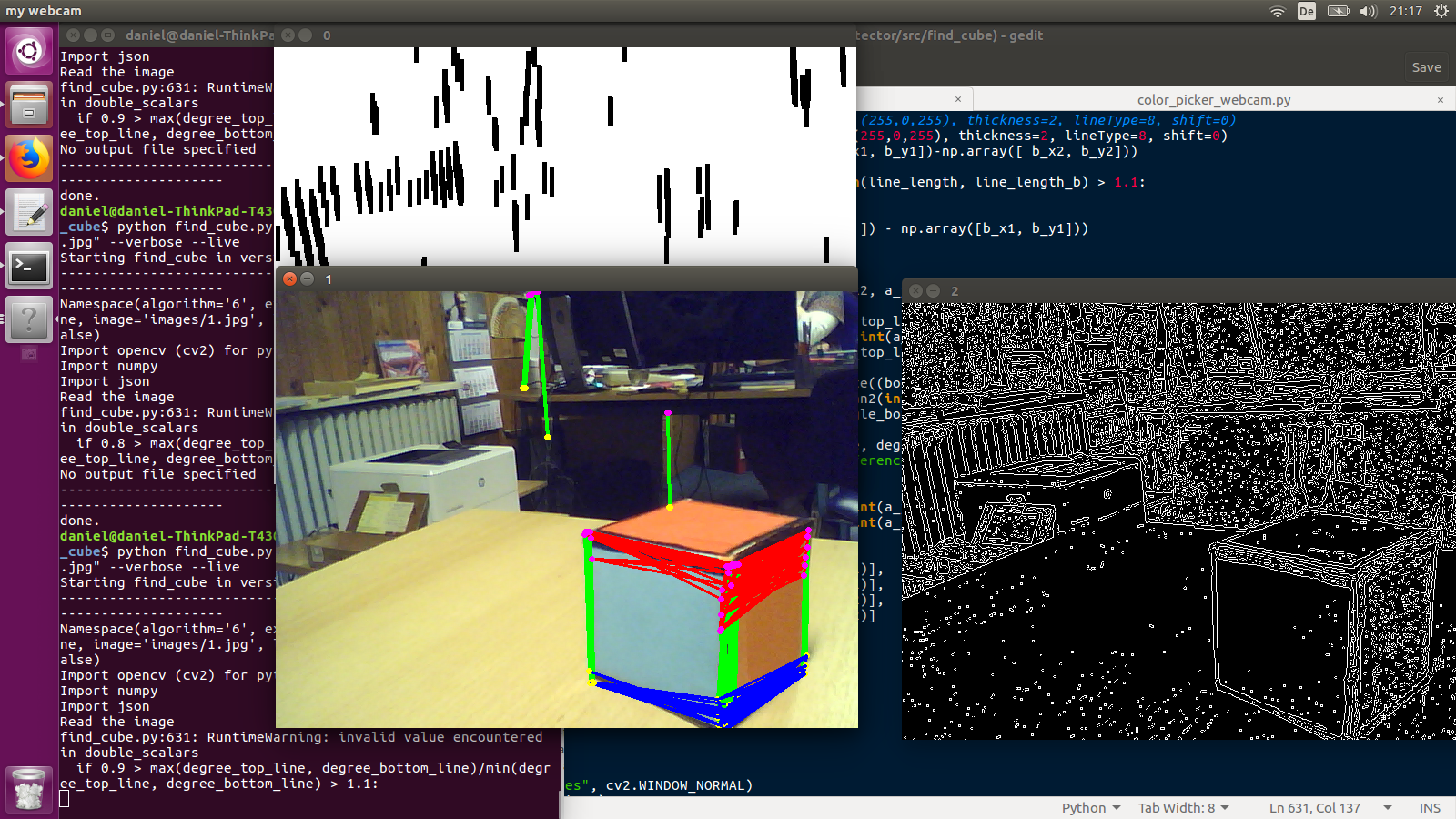As you can see in this image, other lines were detects and had an acceptable length but they were lacking a parallel counterpart within the right range. Therefore only the cubes real edges were connected with red lines.

But the upper lines of the right face of the cube span around 40% of the area.

There are just too many lines and especially too many line ends. That’s why we will cluster them based on their position.

### Cluster the ends of lines with DBSCAN

Initially I was using K-Means as a cluster algorithm. But I soon realized that there is no a priori assumption possible about the K (number of clusters).

That’s why I switched to the (unsupervised) algorithm DBSCAN. Funfact: DBSCAN was created at the same department of the Computer Science faculty of LMU Munich where I worked on my Bachelor thesis.

``````def centeroidnp(arr):
# this method calculates the center of an array of points
length = arr.shape
sum_x = np.sum(arr[:, 0])
sum_y = np.sum(arr[:, 1])
return sum_x/length, sum_y/length

# Promising results of the cluster algorithm
corners = np.array([])
lower_corners = np.array([])
upper_corners = np.array([])

# --------------------------------------------------
# Cluster the lower points
# --------------------------------------------------

# reshape the array to int32 matrix with two columns
vectors = np.int32(lower_points.reshape(-1, 2))

if vectors.any():
# API of DBSCAN from scikit-learn
# http://scikit-learn.org/stable/modules/generated/sklearn.cluster.DBSCAN.html

# Run DBSCAN with eps=30 means that the minimum distance between two clusters is 30px
# and that points within 30px range will be part of the same cluster
db = DBSCAN(eps=75, min_samples=10).fit(vectors)
labels = db.labels_

# Number of clusters in labels, ignoring noise if present.
n_clusters_ = len(set(labels)) - (1 if -1 in labels else 0)

# iterate over the clusters
for i in set(db.labels_):
if i == -1:
# -1 is noise
continue

color = (random.randint(0, 255),random.randint(0, 255),random.randint(0, 255))
index = db.labels_ == i

# draw the members of the cluster
for (point_x, point_y) in zip(vectors[index,0], vectors[index,1]):
cv2.circle(img2,  (point_x, point_y), 5, color, thickness=1, lineType=8, shift=0)

# calculate the centroid of the members
cluster_center = centeroidnp(np.array(zip(np.array(vectors[index,0]),\
np.array(vectors[index,1]))))

# draw the the cluster center
cv2.circle(img2,  cluster_center, 5, color, thickness=10, lineType=8, shift=0)

# store the centroid as corner
corners = np.concatenate((corners, np.array([cluster_center, cluster_center],\
dtype = "uint32")))
lower_corners = np.concatenate((lower_corners,
np.array([cluster_center, cluster_center], dtype = "uint32")))

# --------------------------------------------------
# Cluster the upper points
# = same as with lower points
# --------------------------------------------------

vectors = np.int32(upper_points.reshape(-1, 2))

if vectors.any():
db = DBSCAN(eps=75, min_samples=10).fit(vectors)
labels = db.labels_

n_clusters_ = len(set(labels)) - (1 if -1 in labels else 0)

for i in set(db.labels_):
if i == -1:
continue
color = (random.randint(0, 255),random.randint(0, 255),random.randint(0, 255))
index = db.labels_ == i
for (point_x, point_y) in zip(vectors[index,0], vectors[index,1]):
cv2.circle(img2,  (point_x, point_y), 5, color, thickness=1, lineType=8, shift=0)
cluster_center = centeroidnp(np.array(zip(np.array(vectors[index,0]),\
np.array(vectors[index,1]))))
cv2.circle(img2,  cluster_center, 5, color, thickness=10, lineType=8, shift=0)
corners = np.concatenate((corners, np.array([cluster_center, cluster_center], \
dtype = "uint32")))
upper_corners = np.concatenate((upper_corners, \
np.array([cluster_center, cluster_center], dtype = "uint32")))
``````

The following image shows the plot of the DBSCAN. I used this to calibrate a good epsilon value. In a better version of this program I would have to determine epsilon my other means.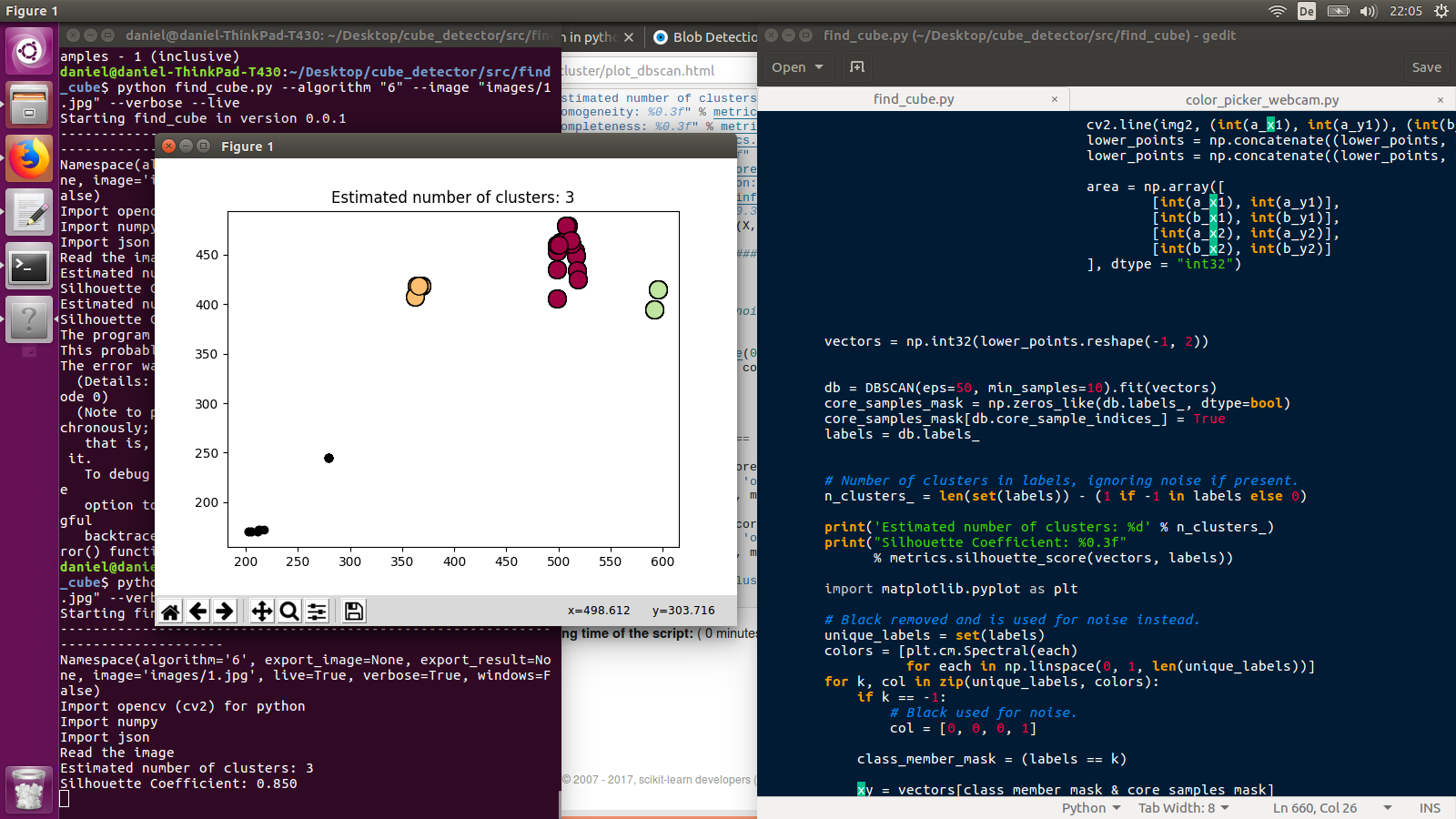The next image shows the assignment of the lower line ends to clusters. Three clusters are detected correctly and the associated points show the outline of the lower corners of the cube.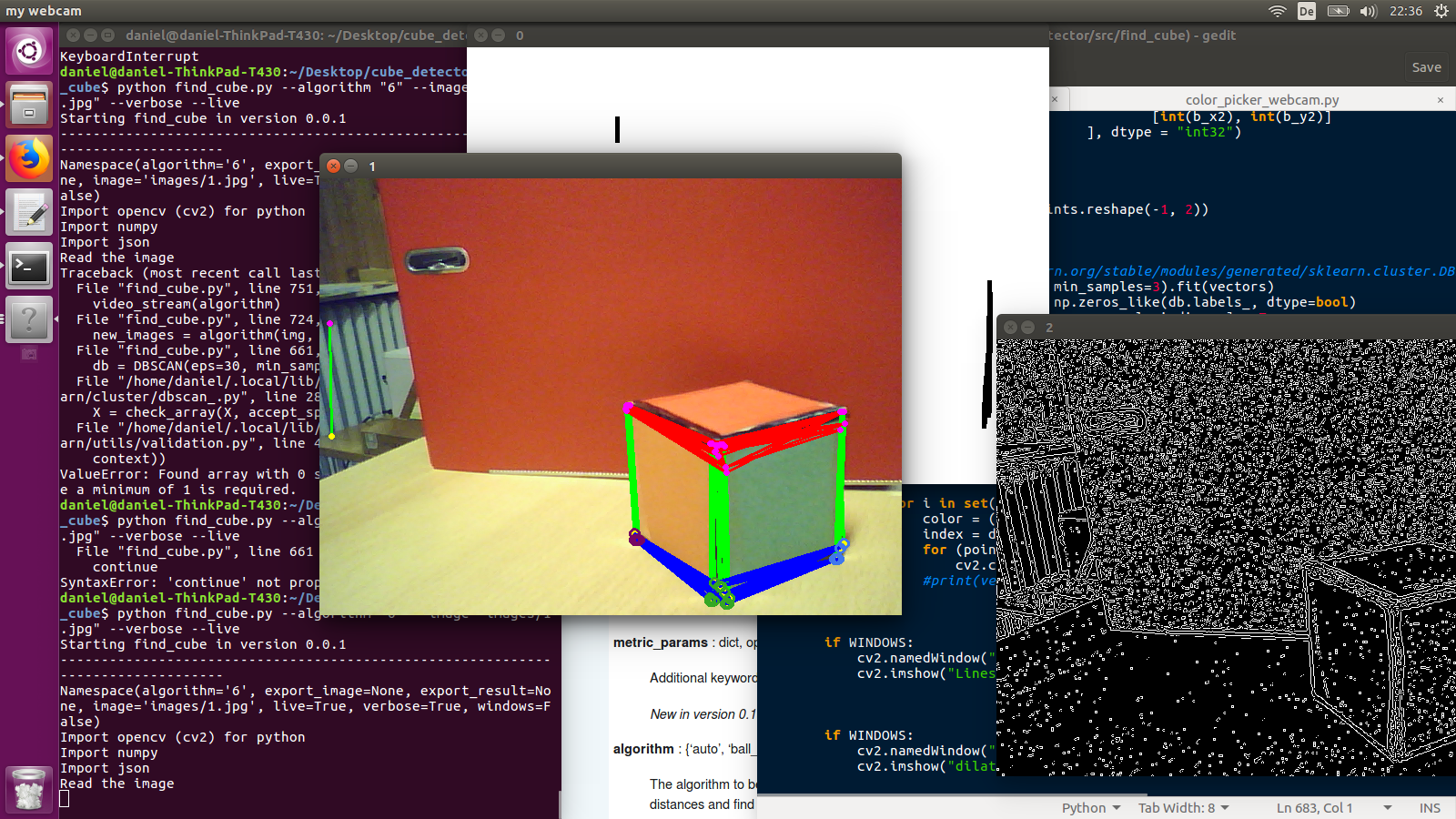## To be continued…

One shall stop at the top and because the reading time of this blog post is already too long I will show the rest of this in a following post.

Coming up:

• tetragon classification by size and dominant background color
• puzzling the tetragons to cubes and picking the right one
• calculate the orientation of the observing camera